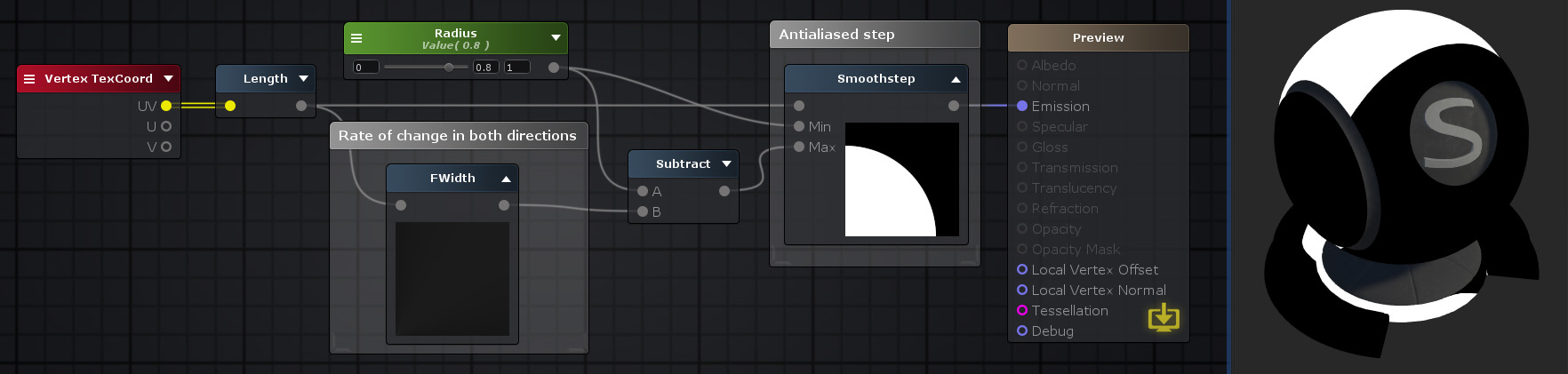## FWidth Node

The Fwith node outputs the absolute value of the partial derivatives of the specified Input value or, in case of vectors, each component value of the input vector. In other words it sums the absolute values of its partial derivatives of the Input value both in horizontal and vertical window space abs(ddx(Input)) + abs(ddy(Input)).Nodes used: Vertex TexCoord, Length, Float, FWidth, Subtract, Smoothstep

Node Parameter Description Default Value
Input Value to be used in the FWidth operation from which the variation will be calculated. Only visible if the respective input port is not connected. 0
Input Port Description Type
Input Value to be used in the FWidth operation from which the variation will be calculated. Float 

1. ^ Port automatically adapts to all connection types except Matrices and Sampler types.